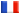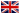# Séminaire de Cryptographie

## Ayoub Otmani### Cryptanalysis of Two McEliece Cryptosystems Based on Quasi-Cyclic Codes

We cryptanalyse here two variants of the McEliece cryptosystem based on quasi-cyclic codes. Both aim at reducing the key size by restricting the public and secret generator matrices to be in quasi-cyclic form. The first variant considers subcodes of a primitive BCH code. The aforementioned constraint on the public and secret keys implies to choose very structured permutations. We prove that this variant is not secure by producing many linear equations that the entries of the secret permutation matrix have to satisfy by using the fact that the secret code is a subcode of a known BCH code. The secret permutation is then extracted by basically solving an over-constrained linear system. This attack has been implemented and in all experiments we have performed the solution space of the linear system was of dimension one and revealed the permutation matrix.

The other variant uses quasi-cyclic low density parity-check codes. This scheme was devised to be immune against general attacks working for McEliece type cryptosystems based on low density parity-check codes by choosing in the McEliece scheme more general one-to-one mappings than permutation matrices. We suggest here a structural attack exploiting the quasi-cyclic structure of the code and a certain weakness in the choice of the linear transformations that hide the generator matrix of the code. This cryptanalysis adopts a polynomial-oriented approach and basically consists in searching for two polynomials of low weight such that their product is a public polynomial. Our analysis shows that with high probability a parity-check matrix of a punctured version of the secret code can be recovered with time complexity $O\left( n3 \right)$ where $n$ is the length of the considered code. The complete reconstruction of the secret parity-check matrix of the quasi-cyclic low density parity-check codes requires the search of codewords of low weight which can be done with about $2^{37}$ operations for the specific parameters proposed.